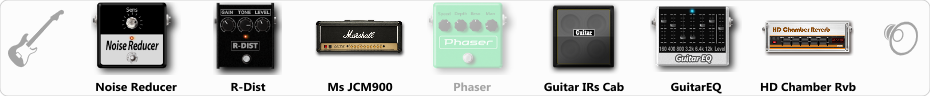# Blessed Are The Sick

Discussion in 'ToneLib-GFX presets' started by mz412, Jun 22, 2019.

1. Blessed Are The Sick

Preset name: Blessed Are The Sick

Morbid Angel inspired OSDM rhythm tone.

Effects chain:Effect: "Noise Reducer" (Dynamics / Filter), active - "yes"
"Sens" = 81
"Mode" = Soft

Effect: "R-Dist" (Overdrive / Distortion), active - "yes"
"Drive" = 41
"Tone" = 84
"Level" = 92

Effect: "Ms JCM900" (Amp simulators), active - "yes"
"Gain" = 41
"Bass" = 61
"Middle" = 38
"Treble" = 75
"Presence" = 71
"Master" = 50
"Output" = 50
"Level (dB)" = 0

Effect: "Phaser" (Modulation / Sfx), active - "no"
"Model" = MXR Phase 90
"Speed" = 1.1
"Depth" = 25
"Reso" = 82
"Freq" = 7.8

Effect: "Guitar IRs Cab" (Cabinets), active - "yes"
"Model" = Marshall 1960b (4x12")
"Mic Position" = Middle
"Mic Distance" = Near
"Low Cut (Hz)" = 89
"Hi Cut (kHz)" = 20.0
"Mix" = 97
"Level (dB)" = 6

Effect: "GuitarEQ" (Dynamics / Filter), active - "yes"
"160 Hz" = 2
"400 Hz" = -3
"800 Hz" = -8
"1.6 kHz" = 5
"3.2 kHz" = 0
"6.4 kHz" = 2
"12 kHz" = 2
"Level (dB)" = 0

Effect: "HD Chamber Rvb" (Reverb), active - "yes"
"Time" = 2.8
"PreLPF" = 38
"PreDelay" = 34
"HiDamp" = 82
"LoGain" = -4.2
"Mix" = 11

Note: You will need to download and install the ToneLib-GFX software to use the preset.

#### Attached Files:

• ###### Blessed_Are_The_Sick.tlgfx
File size:
678 bytes
Views:
3,348
nokturnal and Alvaro Andres like this.